﻿ Vectors

# Vectors

Any physical quantity that has a direction is called a vector. Since all physical quantities have a size that we call magnitude, a vector has both magnitude and direction. Displacement, velocity and force are some of the examples of vectors. If a quantity that does not have direction is called a scalar: length, distance, mass, and speed are scalars.

A vector is drawn as a straight line with an arrow. The length of the line represents the magnitude of the vector and the arrow shows its direction.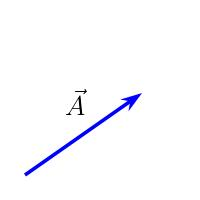Vector A. When writing a vector, we write it as a letter with an arrow over it or as a thick letter.
i.e., vector A is written as $\vec{A}$ or A.
Magnitude of a vector is a positive number, and for vector, $\vec{A}$, magnitude is written with an absolute value symbol, $|\vec A|$ or just $A$, without the arrow.

In an $x$-$y$ co-ordinate system, the angle $\theta$, a vector makes with the $+x$ axis represents the direction of the vector.$\theta$ is the direction of $\vec A$.

### Equal vectors

In a vector, only the magnitude and the direction are important, location is not. If you have two vectors in two different places, but have same magnitude and direction, then they are equal. So, you can move a vector without rotating it and changing its length, still the vector is the same.

### Negative vector

Negative of a vector has same magnitude as the original vector, but has exactly opposite direction. In the following figure, vector $\vec{A}$ is on the left and its negative vector is on the right.

#### Multiplying a vector with a positive number

If you multiply a vector with a positive number, it will change the magnitude of the vector, but not its direction. For example, if you multiply, say $s$, a positive number, with a vector, $\vec{A}$, the magnitude of the vector becomes $sA$.

A vector, $\vec A$ and the vector multiplied with a number $2$ is below. The length (magnitude) of the new vector is twice that of the original vector.But multiplying a negative number changes both the magnitude and the direction. The direction of the new vector will be opposite to the original vector.

If you add (or subtract) two or more vectors, you will get another vector, called resultant vector. There are two ways to add vectors: graphical method and component method (or analytical method). In the graphical method, you will use a ruler and a protractor to draw the vectors and find their resultant vector (magnitude and direction). But using the components method, you can find the magnitude and the direction of the resultant vector without any drawing tools.

### Graphical method

You have two vectors, say $\vec A$ and $\vec B$ and want to add them. Keep the first vector,$\vec A$ and draw (or move) the second vector, $\vec B$ so that its tail (the end with no arrow) is connected to the tip (the end of the vector with the arrow) of the first vector. Now draw a line from the tail of the first vector to the tip of the second vector, which is the resultant vector, $\vec R$.

This graphical method is called tip-to-tail method of vector addition.
In vector addition, order is not important. You can switch $\vec A$ and $\vec B$, i.e., take $\vec B$ as the first vector and $\vec A$ as the second vector, then apply the tip-to-tail method, still you get the same resultant vector:

i.e., $\vec A+\vec B=\vec B+\vec A$.
This is called commutative property of vector addition.

If you have more than two vectors, connect the tail of the second vector to the tip of the first vector, and connect the tail of the third vector to the tip of the second vector and so on. Then draw a line from the tail of the first vector to the tip of the last vector to get the resultant vector.

If you have only two vectors to add, you can get the resultant vector in another way too by forming a parallelogram. Draw the two vectors that you want to add from a common point (where the tail of the two vectors are connected). Taking these two vectors as the adjacent sides, complete a parallelogram. Now draw a diagonal of the parallelogram from the common point. That will be the resultant vector, $\vec R$.

If two vectors point to the same direction or exactly in opposite directions, then we call them parallel vectors.
By the tip-to-tail method, if you add two parallel vectors, $\vec A$ and $\vec B$ of same direction, the resultant, $\vec R$ will have same direction as the original vectors but its magnitude will be the sum of the magnitudes of the two vectors:
Magnitude of $\vec R =$ magnitude of $\vec A+$ magnitude of $\vec B$.
i.e., $R=A+B$.

But, if we add two parallel vectors of opposite directions, then the resultant will have the direction of the larger vector and its magnitude will be the difference of magnitudes of the two vectors.Parallel vectors of opposite directions.
Magnitude of $\vec R$ = magnitude difference of $\vec A$ and $\vec B$.
i.e., $R=|A-B|$.

If you add any two vectors, $\vec A$ and $\vec B$, the magnitude of the resultant will be the minimum when the vectors are opposite to each other, i.e., the minimum magnitude is $|A-B|$. And the resultant magnitude will be the maximum when the vectors are in the same direction, i.e., the maximum magnitude is $A+B$. For example, if we have two vectors of magnitude $15$ and $37$, then the possible magnitude of the resultant is between the numbers $12$ and $52$ as ($|15-37|=12$) and ($15+37=52$). Note that we cannot find the exact magnitude of the resultant vector without knowing the directions of the two vectors.

### Components method

Before going into the components method of vector addition, you need to know what are vector components.
Components of a vector: Assume you have a vector, $\vec A$ that makes an angle $\theta$ with the $+x$-axis. Draw two vectors, one along the $x$ axis, call it $\vec A_x$, and the other along the $y$-axis, call it $\vec A_y$ so that they form a rectangle with $\vec A$ as a diagonal.
The vectors, $\vec A_x$ and $\vec A_y$ are two sides of a parallelogram (i.e., a rectangle) with $\vec A$ as a diagonal from the common point. So, according to the parallelogram method, $\vec A$ is the resultant vector of $\vec A_x$, and $\vec A_y$,

i.e., $\vec A=\vec A_x+\vec A_y$

This tells you that any vector, $\vec A$ can be written as sum of two perpendicular vectors, one along the $x$ axis and the other along the $y$ axis. We call these vectors, component vectors of $\vec A$.

A component vector has only two directions, positive or negative direction. The sign tells the direction of a component vector in the given axis. So, when writing a component vector, we ignore the vector arrow as we did for the velocity and displacement in 1-D motion. So, we write, $A_x$ as the $x$ component of $\vec A$ and $A_y$ is its $y$ component.

Since a vector can be moved without rotating it or changing its length, you can move the component vector $A_y$ to the right side of the rectangle also:

Finding the components of a vector:

To find the components of $\vec A$, take the right angled triangle formed by the vector and its components:

Taking the sine and cosine of $\theta$, we will get

$A_x=A\,cos\theta\:$ and
$A_y=A\,sin\theta$

So, you can find the components of a vector, if you know its magnitude $A$ and direction $\theta$.

You can also go in the reverse. That is if you know the components of a vector, you can find its magnitude and direction. Applying the Pythagorean theorem on the right angled triangle:

$A=\sqrt{A_x^2+A_y^2}$

and taking the tangent of $\theta$,

$tan\theta=\dfrac{A_y}{A_x}$

You can get the angle, $\theta$ by taking the inverse tangent or arc tangent with your calculator.

So far, the vector $\vec A$ we were dealing with was in the first quadrant, where both the components point to the positive directions on their axes, therefore, $A_x$ and $A_y$ were positive. If the vector is in another quadrant, this is not the case. For example, in the figure below, I have a vector, $\vec P$ in the second quadrant, where the $x$ component of $\vec P$ is in the negative $x$ direction, and the $y$ component is in the positive $y$ direction. So, $P_x$ is negative, and $P_y$ is positive.
i.e., $P_x=-P\,cos\theta$ and $P_y=P\,sin\theta$

Sometime, you may be given the angle of the vector from the $y$ axis instead of from the $x$ axis as follows.

If the angle, $\theta$ is from the $y$ axis, then the angle of the vector from the $x$ axis is $90-\theta$. Substituting $90-\theta$ in place of $\theta$ you will get,

$A_x=A\,\cos(90-\theta)=A\,\sin\theta$
$A_y=A\,\sin(90-\theta)=A\,\cos\theta$

Note that the sine and cosine switch if the angle from the $y$ axis is used.Vector addition In the figure, you see that there are two vectors, $\vec A$ and $\vec B$, you need to add them and find the resultant vector. Using the tip-to-tail method, you can draw the resultant vector, $\vec R$ as in the figure. Now, we need to find the magnitude and direction of the resultant vector. Let us draw the $x$ and the $y$ components of $\vec R$, and also that of the vectors, $\vec A$ and $\vec B$.
If you put together and add the $x$ components of $\vec A$ and $\vec B$, you get the $x$ component of $\vec R$: i.e., $R_x=A_x+B_x$
And, if you add the $y$ components of $\vec A$ and $\vec B$, you get the $y$ component of $\vec R$: i.e., $R_y=A_y+B_y$;

The same technique works, if you are adding more than two vectors.
i.e., if you are adding three vectors, $\vec A$, $\vec B$ and $\vec C$,

$\vec R=\vec A+\vec B+\vec C$, then

$R_x=A_x+B_x+C_x$;
$R_y=A_y+B_y+C_y$;
Now you may have a question, what I need to do if I am subtracting one of the vector, say $\vec B$ instead of adding, that is
$\vec R=\vec A-\vec B+\vec C$.
In this case you just need to take the opposite (change the sign) of the $x$ and the $y$ components of $\vec B$, when finding the components of the resultant:

$R_x=A_x-B_x+C_x$;
$R_y=A_y-B_y+C_y$;

Now, you have the $x$ and the $y$ components of $\vec R$, so, you can find its magnitude and direction by the equations:
$R=\sqrt{R_x^2+R_y^2}$
and
$tan\theta=\dfrac{R_y}{R_x}$
Take the inverse tangent to get the direction, $\theta$.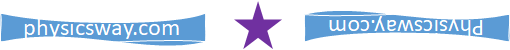Problem: A truck travels 36 km in a direction 32° east of north. Next, it travels 52 km due south. Finally, it travels 64 km in a direction 28° north of west. (a) Determine how far the truck ends up from the starting point, and (b) find the direction of the truck's displacement.

Solution:

There are three displacements for the truck. Each displacement is a vector. Let us label the vectors as $\vec A$, $\vec B$ and $\vec C$. If you draw those vectors, you will get the following figures,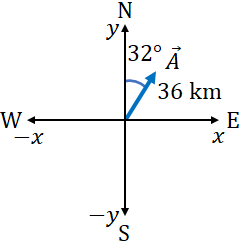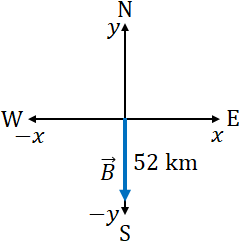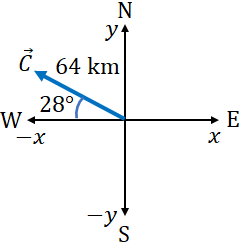Assuming the truck started with the origin, and putting together all the displacement vectors, you get,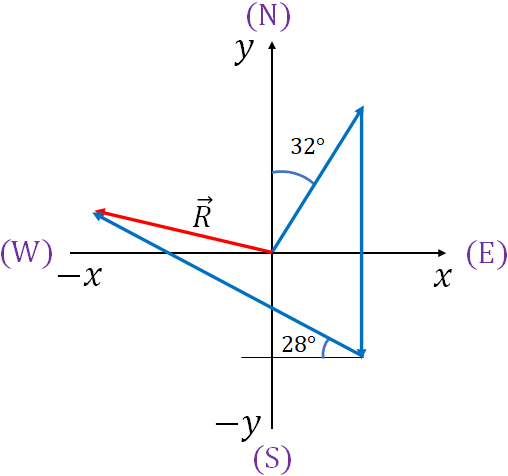The net displacement of the truck is the resultant vector, $\vec R$ drawn from the starting position to the final position of the truck. We need to find how far the truck is from the starting point that is the magnitude, $R$, and the direction, $\theta$ of the resultant vector, which is the direction of the truck's net displacement.

First step is, you need to find the $x$ and the $y$ components of all the three vectors $\vec A$, $\vec B$ and $\vec C$. Then add all the $x$ components that will give you the $x$ component of the resultant, $R_x$, and add all the $y$ components, you will get the $y$ component of the resultant, $R_y$. From $R_x$ and $R_y$ you can find $R$ and $\theta$.

Take the vectors one by one, draw and find their $x$ and $y$ components.

$\underline{\vec A}$:Magnitude of the vector is $A= 36 km$.

The components, $A_x$ and $A_y$ both are positive as $A_x$ is in the $+x$ direction and $A_y$ is in the $+y$ direction. And the angle from the $y$ axis is given, therefore

$A_x=+A \sin \theta = 36km \sin 32= 19.08 km$

$A_y=+A \cos \theta = 36km \cos 32= 30.53 km$

$\underline{\vec B}$: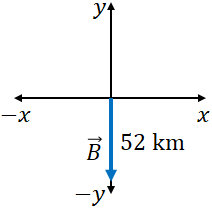Magnitude of the vector is $B= 52 km$. The vector is on the $y$ axis, so its $x$ component is zero. Further, the vector is on the negative $y$ axis, so the $y$ component is negative:

$B_x=0$

$B_y=-B=-52km$

$\underline{\vec C}$: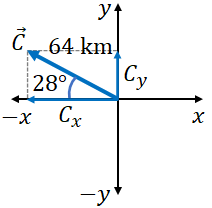Magnitude, $C= 64 km$; $\theta = 28^\circ$ from the $x$ axis.

$C_x$ is negative as it is on the negative $x$ axis, $C_y$ is positive as it is on the positive $y$ axis. Therefore,

$C_x=-C \cos\theta= -64km \cos 28=-56.51 km$

$C_y=+C \sin\theta= 64km \sin 28=30.05 km$

Adding the $x$ components:

$R_x=A_x+B_x+C_x$

$=19.08 km+0-56.51km$

$=-37.43km$

Adding the $y$ components:

$R_y=A_y+B_y+C_y$

$=30.53 km-52 km+30.05 km$

$=8.58 km$

Magnitude of the resultant is

$R=\sqrt{R_x^2+R_y^2}$

$=\sqrt{(-37.43)^2+(8.58)^2} \,km$

$=38.4 km$

Now, find $\theta$ by using the equation:

$tan \theta = \dfrac{R_y}{R_x}$

$=\dfrac{8.58}{-37.43}$

$=-0.229$

or

$\theta = -13^\circ$

$\theta$ is negative means, the vector, $\vec R$ should be below the positive $x$ axis or above the negative $x$ axis. To find which one, draw the $x$ and the $y$ components of $\vec R$ as follows. From the components draw the $\vec R$ by forming a rectangle. $\vec R$ is above the negative $x$ axis (this is what you already got, from a picture above).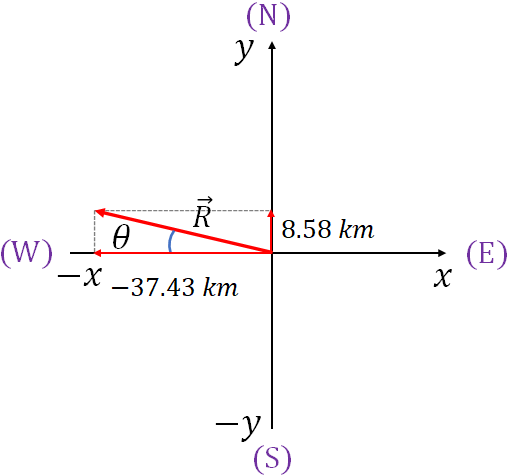Since the negative direction is the east, and the vector is 13° above the east and towards the north, the direction of the tructk's displacement is 13° north of east.You could notice that, an important property of vector components is that, they are independent of each other. If you change one component of a vector it does not affect the other component. i.e, changing the $x$ component of a vector does not change its $y$ component and vice versa.